# Evaluating Functions

Introduction

Evaluating a function means finding the value of f(x) =… or y =… that corresponds to a given value of x. To do this, simply replace all the x variables with whatever x has been assigned. For example, if we are asked to evaluate f(4), then x has been assigned the value of 4.

When we have a function in formula form, it is usually a simple matter to evaluate the function. For example, the function f(x)=5−3x2 f ( x ) = 5 − 3 x 2 can be evaluated by squaring the input value, multiplying by 3, and then subtracting the product from 5.

At the end of the lesson, the learner is able to evaluate functions and solve problems involving functions.

Process

Example 1.  Evaluate the following functions at x = 1.5:

(g) f(x) = 2x + 1
(h) q(x) =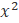– 2x + 2

(i) g(x) =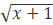(j) r(x) =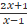(k) F(x) =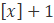, where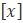is the greatest integer in function.

Solution. Substituting 1.5 for x in the functions above, we have

(a) f(x) = 2(1.5) + 1 =  4

(b) q(x) =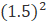– 2(1.5) + 2 = 2.25 – 3 + 2 = 1.25

(c) g(x) =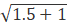=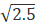(d) r(x) =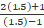=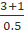= 8

(e) F(1.5) =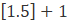= 1 + 1 = 2

Example 2.  Find g(-4) and r(10 where g and r are as defined in the previous example.

Solution. This is not possible because - 4 is not in the domain of g(x) and 1 is not in the domain of r 9(x)

Example 3.  Evaluation the following functions, where f and q are as defined in  Example 1.

(a) f (3x - 1 )                                      (b) (2x + 3)

Solution.
(a)  ( 3x - 1 ) = 2 (3x - 1) + 1 = 6x - 2 + 1 = 6x - 1
(b) ( 2x + 3 ) =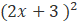- 2(2x + 3 ) + 2 = (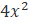+ 12x + 9 ) - 4x - 6 + 2
=+ 8x  + 5

Solved Examples.

1. Evaluate the following functions at x=3.

(a) f (x) = x - 3

(b) g (x) =- 3x + 5

(c) h (x) =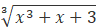(d) p (x) =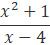(e) f (x) = lx - 5l where lx -5l means the absolute value of x-5.

Solution.

(a) f (3) = 3 - 3 = 0

(b) g (x) =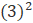- 3(3) + 5 = 9 - 9 +5 = 5

(c) h (x) =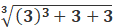​​=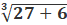=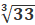(d) p (x) =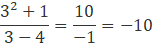(e) f (x) =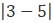=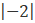= 2

2. For what values of x can we not evaluate the function f(x) =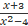?

Solution.

The domain of the function is given by { x: x  € ℝ, x ≠ ±2}. Since 2 and -2 are not in t he domain, we cannot evaluate the function at x = -2,2.

3. Evaluate ( a + b ) where  f (x) =- 3x.

Solution.

( a + b )  = 4 ( a + b ) =4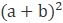- ( a + b ) = 4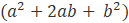- 3a - 3b =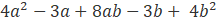4. Suppose that s (T) is the top speed ( in km per hour) of a runner when the temperature is T degree Celsius. Explain what the statements s(15) = 12 and s(30) = 10 mean.

Solution. The first equation means that when the temperature is 15ºC, then the top speed of a runner is 12 km per hour. However, when temperature rises to 30ºC, the top speed is reduced to 10km per hour.

5. The velocity V (in m/s) of a ball thrown upward t seconds after the ball was thrown is given by V(t) = 20 - 9.8t. Calculate V(0) and V(1), and explain what these results mean.

Solution. V(0) = 20 - 9.8(0) = 20 and V(1) =20 - 9.8(1) = 10.2. These results indicate that the initial velocity of the ball is 20 m/s. After 1 second, the ball is travelling more slowly, at 10.2 m/s.

Evaluation

## Supplementary Exercises.

1. Evaluate the following functions at x = -4.

(a) f(x) =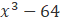(b) g(x) =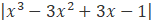(c) r(x) =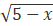(d) q(x) =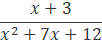2. Given f(x) =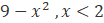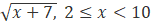, give the values of the following :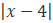, x ≥ 10

(a) f(2)
(b) f(12.5)
(c) f(-3)
(d) f(5)
(e) f(1.5)

3.   Given f (x)=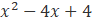. Solve for

(a) f(3)
(b) f(x + 3)
is f (x +3) the same as f ( x) + f (3)?

4. A computer shop chargers P20.00 per hour (or a fraction of an hour) for the first two hours and an additional P10.00 per hour for each succeeding hour. Find how much you would pay if you use one of their computer for:

(a) 40 minutes
(b) 3 hours
(c) 150 minutes.

5. Under certain circumstances, a rumor spreads according to the equation

p(t)=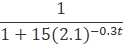where p(t) is the proportion of the population that knows the rumor (t) days after the rumor started. Find p(4) and p(10), and interpret the results.

Credits